This book is archived and will be removed July 6, 2022. Please use the updated version.

Sources of Magnetic Fields

# 80 Magnetic Field of a Current Loop

### Learning Objectives

By the end of this section, you will be able to:

• Explain how the Biot-Savart law is used to determine the magnetic field due to a current in a loop of wire at a point along a line perpendicular to the plane of the loop.
• Determine the magnetic field of an arc of current.

The circular loop of (Figure) has a radius R, carries a current I, and lies in the xz-plane. What is the magnetic field due to the current at an arbitrary point P along the axis of the loop?

Determining the magnetic field at point P along the axis of a current-carrying loop of wire.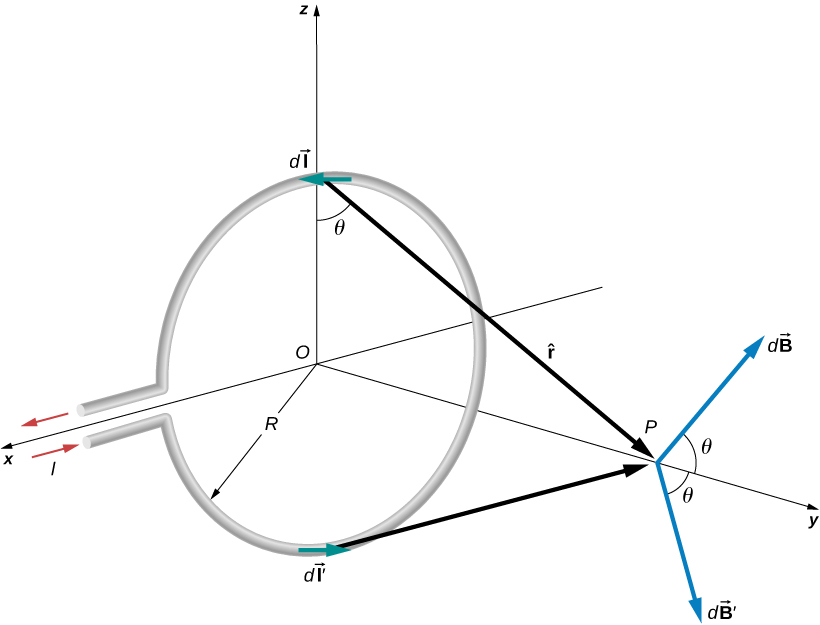We can use the Biot-Savart law to find the magnetic field due to a current. We first consider arbitrary segments on opposite sides of the loop to qualitatively show by the vector results that the net magnetic field direction is along the central axis from the loop. From there, we can use the Biot-Savart law to derive the expression for magnetic field.

Let P be a distance y from the center of the loop. From the right-hand rule, the magnetic field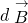at P, produced by the current element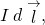is directed at an angle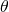above the y-axis as shown. Since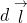is parallel along the x-axis and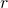is in the yz-plane, the two vectors are perpendicular, so we have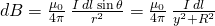where we have used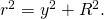Now consider the magnetic field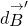due to the current element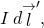which is directly opposite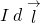on the loop. The magnitude ofis also given by (Figure), but it is directed at an anglebelow the y-axis. The components ofandperpendicular to the y-axis therefore cancel, and in calculating the net magnetic field, only the components along the y-axis need to be considered. The components perpendicular to the axis of the loop sum to zero in pairs. Hence at point P: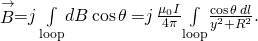For all elementson the wire, y, R, and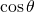are constant and are related by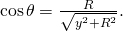Now from (Figure), the magnetic field at P is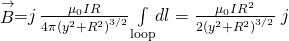where we have used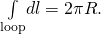As discussed in the previous chapter, the closed current loop is a magnetic dipole of moment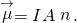For this example,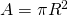and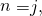so the magnetic field at P can also be written as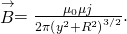By setting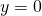in (Figure), we obtain the magnetic field at the center of the loop: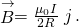This equation becomes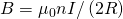for a flat coil of n loops per length. It can also be expressed as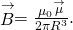If we consider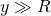in (Figure), the expression reduces to an expression known as the magnetic field from a dipole: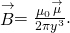The calculation of the magnetic field due to the circular current loop at points off-axis requires rather complex mathematics, so we’ll just look at the results. The magnetic field lines are shaped as shown in (Figure). Notice that one field line follows the axis of the loop. This is the field line we just found. Also, very close to the wire, the field lines are almost circular, like the lines of a long straight wire.

Sketch of the magnetic field lines of a circular current loop.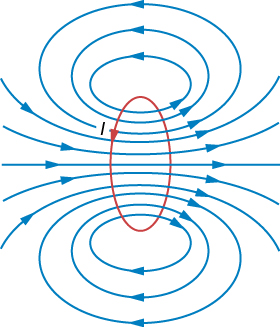Magnetic Field between Two Loops Two loops of wire carry the same current of 10 mA, but flow in opposite directions as seen in (Figure). One loop is measured to have a radius of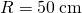while the other loop has a radius of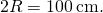The distance from the first loop to the point where the magnetic field is measured is 0.25 m, and the distance from that point to the second loop is 0.75 m. What is the magnitude of the net magnetic field at point P?

Two loops of different radii have the same current but flowing in opposite directions. The magnetic field at point P is measured to be zero.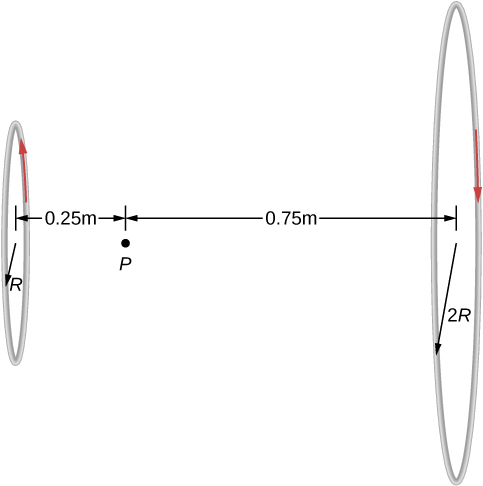Strategy The magnetic field at point P has been determined in (Figure). Since the currents are flowing in opposite directions, the net magnetic field is the difference between the two fields generated by the coils. Using the given quantities in the problem, the net magnetic field is then calculated.

Solution Solving for the net magnetic field using (Figure) and the given quantities in the problem yields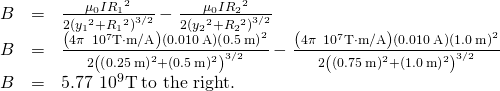Significance Helmholtz coils typically have loops with equal radii with current flowing in the same direction to have a strong uniform field at the midpoint between the loops. A similar application of the magnetic field distribution created by Helmholtz coils is found in a magnetic bottle that can temporarily trap charged particles. See Magnetic Forces and Fields for a discussion on this.

Check Your Understanding Using (Figure), at what distance would you have to move the first coil to have zero measurable magnetic field at point P?

0.608 meters

### Summary

• The magnetic field strength at the center of a circular loop is given by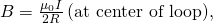where R is the radius of the loop. RHR-2 gives the direction of the field about the loop.

### Conceptual Questions

Is the magnetic field of a current loop uniform?

What happens to the length of a suspended spring when a current passes through it?

The spring reduces in length since each coil with have a north pole-produced magnetic field next to a south pole of the next coil.

Two concentric circular wires with different diameters carry currents in the same direction. Describe the force on the inner wire.

### Problems

When the current through a circular loop is 6.0 A, the magnetic field at its center is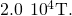What is the radius of the loop?

0.019 m

How many turns must be wound on a flat, circular coil of radius 20 cm in order to produce a magnetic field of magnitude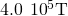at the center of the coil when the current through it is 0.85 A?

A flat, circular loop has 20 turns. The radius of the loop is 10.0 cm and the current through the wire is 0.50 A. Determine the magnitude of the magnetic field at the center of the loop.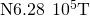A circular loop of radius R carries a current I. At what distance along the axis of the loop is the magnetic field one-half its value at the center of the loop?

Two flat, circular coils, each with a radius R and wound with N turns, are mounted along the same axis so that they are parallel a distance d apart. What is the magnetic field at the midpoint of the common axis if a current I flows in the same direction through each coil?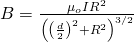For the coils in the preceding problem, what is the magnetic field at the center of either coil?